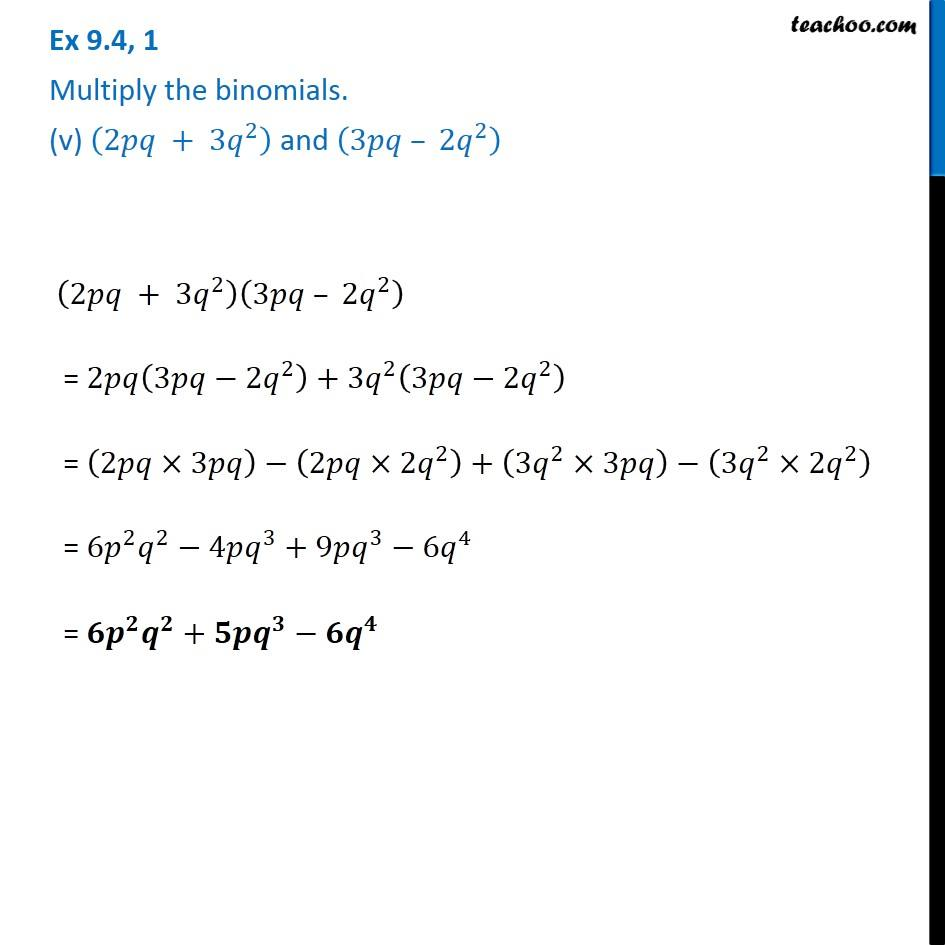Subscribe to our Youtube Channel - https://you.tube/teachoo

1. Chapter 9 Class 8 Algebraic Expressions and Identities
2. Concept wise
3. Multiplication of Polynoimals by Polynomials

Transcript

Ex 9.4, 1 Multiply the binomials. (v) (2𝑝𝑞 + 3𝑞^2 ) and (3𝑝𝑞 – 2𝑞^2 ) (2𝑝𝑞 + 3𝑞^2 )(3𝑝𝑞 – 2𝑞^2 ) = 2𝑝𝑞(3𝑝𝑞−2𝑞^2 )+3𝑞^2 (3𝑝𝑞−2𝑞^2 ) = (2𝑝𝑞×3𝑝𝑞)−(2𝑝𝑞×2𝑞^2 )+(3𝑞^2×3𝑝𝑞)−(3𝑞^2×2𝑞^2 ) = 6𝑝^2 𝑞^2−4𝑝𝑞^3+9𝑝𝑞^3−6𝑞^4 = 𝟔𝒑^𝟐 𝒒^𝟐+𝟓𝒑𝒒^𝟑−𝟔𝒒^𝟒

Multiplication of Polynoimals by Polynomials

About the AuthorDavneet Singh
Davneet Singh is a graduate from Indian Institute of Technology, Kanpur. He has been teaching from the past 9 years. He provides courses for Maths and Science at Teachoo.